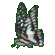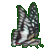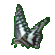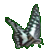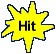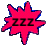Math Help - Fun Arithmetic Help - Perimeter Word Problems - Game Tips:

- There are 20 random questions on Perimeters of quadrilaterals, triangles, parallelograms and circles.

- The Perimeter of a quadrilateral is found by adding up the lengths of its 4 sides.
- If the opposite sides are equal or parallel as in a rectangle or a parallelogram,
then this simply becomes P = 2(long sides)+2(short sides).

- The Perimeter of a triangle is found by adding up the lengths of its 3 sides, P = a+b+c.

- The Perimeter (Circumference) of a circle is found by multiplying its diameter times π .
C = πD where π = 3.14 approx.

- Your Game Score is reduced by the number of butterfly hits.

- To slow the game speed repeat tap/click on the word Slider.
- To increase the game speed repeat tap/click on the word Math.
- Speed can also be adjusted with a keyboard's - and + keys.

- Refresh/Reload the web page to restart the game.